#### Chapter 11 Co-ordinate Geometry R.D. Sharma Solutions for Class 9th MCQ's

Multiple Choice Questions

Q1. Mark the correct alternative in each of the following:
The point of intersect of the coordinate axes is
(a) ordinate
(b) abscissa
(d) origin

Solution

As we know that:
The distance of a point from y−axis is called its x−coordinate or abscissa.
The distance of a point from x−axis is called its y−coordinate or ordinate.
The coordinate axes divide the plane into four equal parts which are known as quadrants.
The point of intersection of the coordinate axes is called the origin and the coordinates of origin are (0, 0).
Example is shown in the graphThus correct answer is d .

2. The abscissa and ordinate of the origin are
(a) (0, 0)
(b) (1, 0)
(c) (0, 1)
(d) (1, 1)

Solution

As we know that:
The distance of a point from y-axis is called its x-coordinate or abscissa.
The distance of a point from x-axis is called its y-coordinate or ordinate.
The coordinate axes divide the plane into four equal parts which are known as quadrants.
The point of intersection of the coordinate axes is called the origin and the coordinates of origin are (0, 0).The origin is shown in the graph
Thus the correct answer is (a).

3. The measure of the angle between the coordinate axes is
(a) 0°
(b) 90°
(c) 180°
(d) 360°

Solution

As we know that x-axis and y-axis intersect to each other at point O and perpendicular to each other. So, the angle between the coordinate axes is 90°.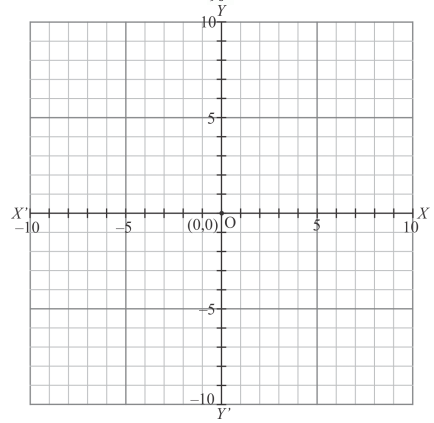Thus the correct answer is (b).

4. A point whose abscissa and ordinate are 2 and −5 respectively, lies in

Solution

As shown in graph that a point whose abscissa and ordinate are 2 and -5 respectively lies in the fourth quadrant.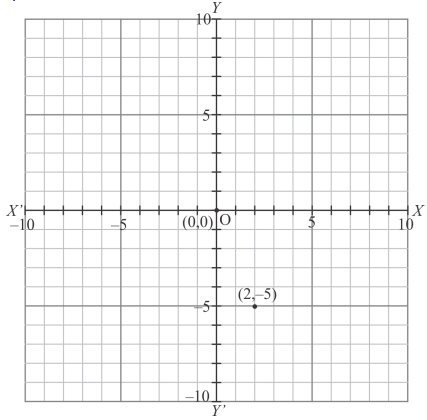Thus the correct answer is (d).

5. Points (-4, 0) and (7, 0) lie
(a) on x-axis
(b) y-axis

Solution

Let the points P and Q whose coordinates are (-4,0) and (7, 0) respectively. Locate the points and you will see that they lie on x-axis.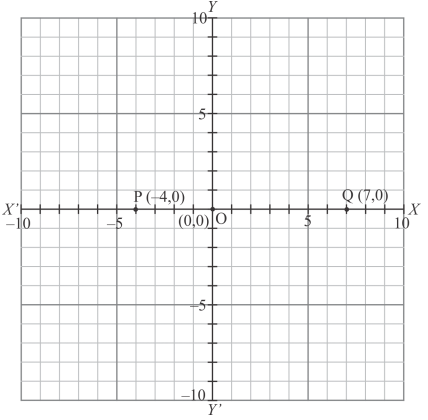Thus the correct answer is (a)

6. The ordinate of any point on x-axis is
(a) 0
(b) 1
(c) −1
(d) any number

Solution

We know that the y-coordinates of every point on x-axis are zero. So, the coordinates of any point on the x-axis are of the form (x, 0)
Thus the correct answer is (a).

7. The abscissa of any point on y-axis is
(a) 0
(b) 1
(c) −1
(d) any number

Solution

We know that the x-coordinate of every point on y-axis is zero. So, the coordinates of any point on the x-axis are of the form (0, y).
Thus the correct answer is (a).

8. The abscissa of a point is positive in the

Solution

The signs of coordinates  (x, y) of a point in various quadrants are shown in the following graph: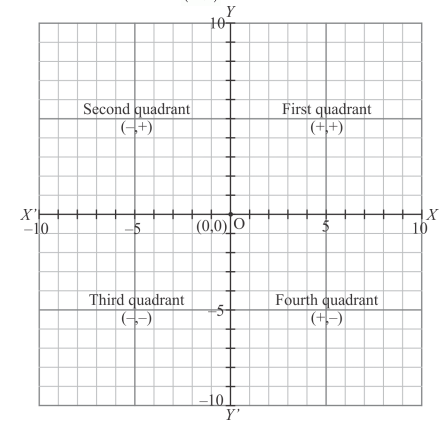Thus the correct answer is (d)

9. A point whose abscissa is −3 and ordinate 2 lies in

Solution

As we know that
In the first quadrant :  x >0, y>0
In the second quadrant:  x<0, y>0
In the third quadrant:  x<0, y<0
In the fourth quadrant : x>0, y<0The point whose abscissa is -3 which is negative and ordinate 2 is positive, so this point lies in the second quadrant.
Thus the correct answer is (b).

10. Two points having same abscissas but different ordinate lie on
(a) x-axis
(b) y-axis
(c) a line parallel to y-axis
(d) a line parallel to x-axis

Solution

Let the points P(3, 5) and Q(3, 2) having the same abscissa but different ordinates be shown in the graph given below: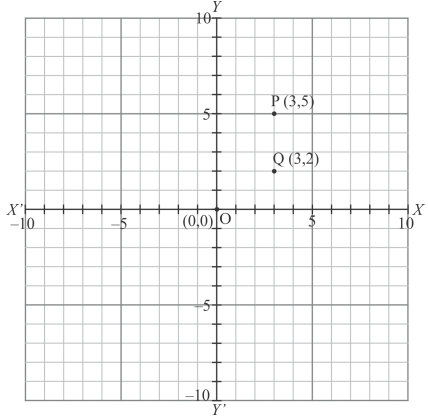Fig: (location of two considered points)
And these points lie on a line parallel to y-axis
Thus the correct answer is (c).

11. The perpendicular distance of the point P (4, 3) from x-axis is
(a) 4
(b) 3
(c) 5
(d) none of these

Solution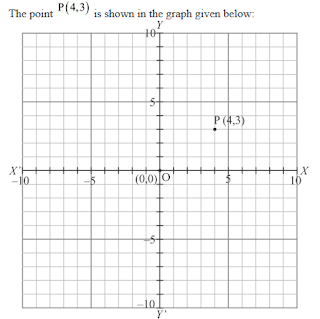Thus the perpendicular distance of the point  P (4, 3) from x-axis is 3 units. Thus the correct answer is (b)

12. The perpendicular distance of the P (4,3)  from y-axis is
(a) 4
(b) 3
(c) 5
(d) none of these

Solution

The point P (4, 3) is shown in the graph given below:Thus the perpendicular distance of the point P (4, 3)from y-axis is 4.
Thus the correct answer is (a).

13. The distance of the point P (4, 3) from the origin is
(a) 4
(b) 3
(c) 5
(d) 7

Solution

The point P(4, 3) is shown in the graph given below:14. The area of the triangle formed by the points A(2,0) B(6,0) and C(4,6) is

(a) 24 sq. units
(b) 12 sq. units
(c) 10 sq. units
(d) none of these

Solution

Given that points  A(2, 0) , B(6, 0) and C(4, 6) , form a triangle which is shown in the figure. We are asked to find the area of the triangle ΔABC.15. The area of the triangle formed by the points P (0, 1), Q (0, 5) and R (3, 4) is
(a) 16 sq. units
(b) 8 sq. units
(c) 4 sq. units
(d) 6 sq. units

Solution

Given that the points P(0, 1) , Q(0, 5) and  R(3, 4) form a triangle.
We are asked to find the area of the triangle ΔPQR which is shown in the figure.